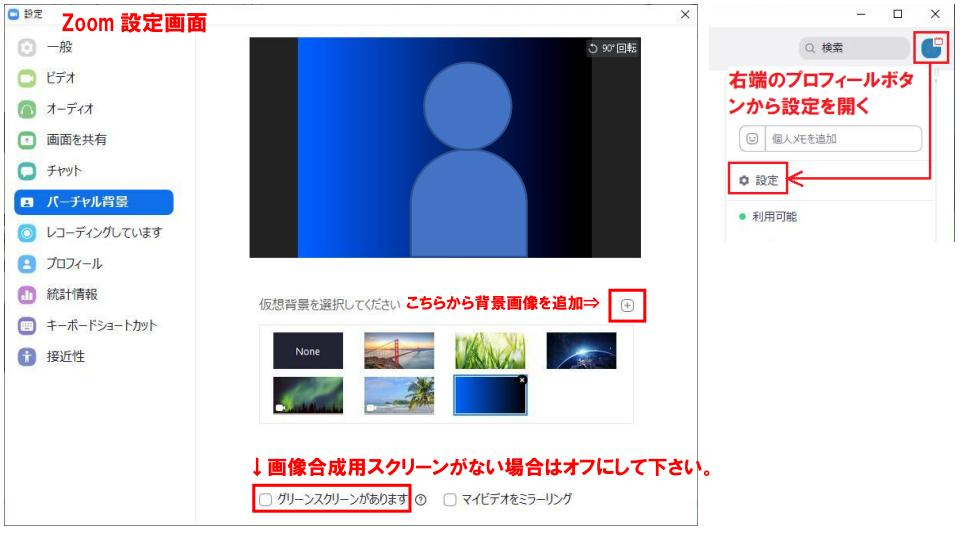# 常用対数の近似値の算出

$z=x^y$での$y$を知りたい場合に$y=\log_{x}z$と書く。

（※自然対数は対数の底がeのもの。${\rm e}^x$は微分しても${\rm e}^x$のままなので便利）

$\log_{10} 1 = 0, (10^0=1)$
$\log_{10} 2 \fallingdotseq 0.3$

$\log_{10} 2^{10}=\log_{10} 1024\fallingdotseq \log_{10} 1000=\log_{10}10^3=3\log_{10}10=3\cdot 1=3$

$\Rightarrow 10\log_{10}2\fallingdotseq3 \Rightarrow \log_{10}2\fallingdotseq \displaystyle\frac{3}{10}=0.3$

$\log_{10}3\fallingdotseq 0.475, (10^{0.5}=\sqrt{10}\fallingdotseq \sqrt{9}=3)$

$\log_{10}3^4=\log_{10}(9\cdot 9)=\log_{10}{81}\fallingdotseq \log_{10}80=\log_{10}(2^3\cdot 10) \Rightarrow 3\log_{10}2 + \log_{10}10 \fallingdotseq 3\cdot 0.3 + 1 = 1.9$
$\Rightarrow 4\log_{10}3\fallingdotseq 1.9 \Rightarrow \log_{10}3\fallingdotseq\displaystyle\frac{1.9}{4}=0.475$

$\log_{10}4\fallingdotseq 0.6$

$\log_{10}4=\log_{10}2^2=2\log_{10}2\fallingdotseq 2\cdot0.3=0.6$

$\log_{10}5\fallingdotseq 0.7$

$\log_{10}5=\log_{10}\displaystyle\frac{10}{2}=\log_{10}10-\log_{10}2\fallingdotseq 1-0.3 = 0.7$

$\log_{10}6\fallingdotseq 0.775$

$\log_{10}6=\log_{10}(2\cdot 3)=\log_{10}2 + \log_{10}3\fallingdotseq 0.3 + 0.475 = 0.775$

$\log_{10}7=0.85$

$\log_{10}7^2=\log_{10}49\fallingdotseq \log_{10}50 = \log_{10}(5\cdot10) = \log_{10}5+\log_{10}10\fallingdotseq 0.7+1$
$\Rightarrow 2\log_{10}7\fallingdotseq 1.7 \Rightarrow \log_{10}7\fallingdotseq \displaystyle\frac{1.7}{2}=0.85$

$\log_{10}7^4=\log_{10}2401\fallingdotseq \log_{10}2400 = \log_{10}(24\cdot100) = \log_{10}8+\log_{10}3+\log_{10}100$
$=\log_{10}2^3+\log_{10}3+\log_{10}10^2=3\log_{10}2+\log_{10}3+2\log_{10}10\fallingdotseq 3\cdot 0.3 + 0.475 + 2\cdot 1.0=3.375$
$\Rightarrow4\log_{10}7=3.375\Rightarrow\log_{10}7\fallingdotseq 3.375/4=0.84375\fallingdotseq 0.844$

$\log_{10}8\fallingdotseq 0.9$

$\log_{10}8=\log_{10}2^3=3\log_{10}2\fallingdotseq 3\cdot 0.3 = 0.9$

$\log_{10}9\fallingdotseq 0.95$

$\log_{10}9=\log_{10}3^2=2\log_{10}3\fallingdotseq 2\cdot 0.475=0.95$

$\log_{10}9^7=\log_{10}4782969\fallingdotseq \log_{10} 4800000 = \log_{10} (6\cdot 8\cdot 10^5) = \log_{10} 6 + \log_{10} 8 + \log_{10} 10^5$
$= 0.775 + 0.9 + 5.0=6.675$
$\Rightarrow 7\log_{10}9=6.675 \Rightarrow \log_{10}9 \fallingdotseq 6.675/7=0.9537\cdots\fallingdotseq 0.954$

$\log_{10}10=1, (10^1=10)$

おまけ：$\log_{10}11\fallingdotseq 1.04$

$\log_{10}11^5=\log_{10}161051\fallingdotseq \log_{10}160000=\log_{10}2^4\cdot10^4=4(\log_{10}2+\log_{10}10)\fallingdotseq 4(0.3+1.0)$
$\Rightarrow 5\log_{10}11=4\cdot1.3\Rightarrow \log_{10}11\fallingdotseq \displaystyle\frac{4}{5}1.3=0.8\cdot 1.3=1.04$

この近似をプロットすると下図のようになる。$\log_{10}7$と$\log_{10}9$は他に比べて誤差が大きいので、高精度版を赤色として示した。1 2 3 4 5 6 7 8 9 10

## 観察

• 1～10で、$\log_{10}$は0～1になる。
• 3で$\log_{10}$は約0.45で、ざっくり半分に到達する。
• 2は$\log_{10}$は約0.3で、$4=2^2$, $8=2^3$だと$\log_{10}$はそれぞれ2,3倍の約0.6,0.9となる。8の際に既にほぼ1に近いことに注意。
• 5は10/2で、$\log_{10}$は$1-\log_{10}2$となるので約0.7。1と10の両端側からの距離でいうと2の場合と同じで約0.3。
• 倍になると、対数では同じだけ増加する。
例）
$\log_{10}2=0.3\stackrel{\times 2}{\longrightarrow}\log_{10}4=0.6\stackrel{\times 2}{\longrightarrow}\log_{10}8=0.9$,
$\log_{10}3=0.475\stackrel{\times 3}{\longrightarrow}\log_{10}9=0.95$,
$\log_{10}5=0.7\stackrel{\times 2}{\longrightarrow}\log_{10}10=1.0$
$\log aM=\log a + \log M$より、$a$倍されていれば、$\log a$分だけ増加する（割れば$\log a$だけ減少する）。$\rightarrow$掛け算・割り算が同じ距離の変化になる。

• 2で割ると$\log_{10}$では0.3減少するので、これを用いると

$\log_{10}3/2(=\log_{10}1.5)\fallingdotseq 0.475-0.3=0.175$
$\log_{10}5/2(=\log_{10}2.5)\fallingdotseq 0.7-0.3=0.4$
$\log_{10}7/2(=\log_{10}3.5)\fallingdotseq 0.85-0.3=0.55$
$\log_{10}9/2(=\log_{10}4.5)\fallingdotseq 0.95-0.3=0.65$

となる。1/2も求めてみると、

$\log_{10}1/2(=\log_{10}0.5)\fallingdotseq 0-0.3=-0.3$

となる。

$\log_{10}0.5=\log_{10}\displaystyle\frac{5}{10}=\log_{10}5-\log_{10}10\fallingdotseq 0.7-1=-0.3$

でも結果は同様。

# Zoom：バーチャル背景用の単色系の背景画像を作った

バーチャル背景の機能も優秀でスクリーンなしでも背景を置き換えてくれるようだが、そのままだと元から用意されている背景画像が、宇宙やらビーチやら壮大、軽快過ぎてちょっと会議等の状況にそぐわない。とはいえ、単なる単色だけだと味気ないので、ほんのりグラデーションを入れた。左側を少し明るめで右側を暗めにするほうがいいみたい。

グラデーション生成にはInkscapeを使った。

バーチャル背景の設定は、画面上部右端のプロフィールボタンから、設定⇒バーチャル背景と選び、任意の画像を指定する。このとき、設定下部の「グリーンスクリーンがあります。」がオンとなっていると、合成用のスクリーンがある前提での動作になってしまうので、スクリーンを使わない場合はこれは外しておく。

Zoomは脆弱性等が問題山積みになっているようだが、今のところ一択ツール。なんとか修正・改善されて無事に使えますように…。

どこまで使い物になるか不明だが、同種のオープンソースツールもある。

あと、Zoomの紹介動画をいくつか。

# A6とハガキの用紙サイズの違い

A4用紙の折り方か、切り方を間違えたのかと思ったが、やっぱり違う。

と思ったのだが、ハガキのサイズは万国共通（万国郵便連合）で決まっているとのこと。

サイズを入れた比較図をパワポでのマウス操作で作ってもみたが、どうせならと手入力SVGで作成してみた。ほぼ、単に四角を２つ並べるだけだから（初心者でも）簡単だろうと思ったらけっこう手こずったわ…。（かくして、この記事に続く…⇒MDN文書の翻訳

[おまけ]
あと、WordPressにSVGをアップロードしようとしたらセキュリティ関連で蹴られて、プラグインを入れて対応した。プラグインは探すといくつか出てきたのだが、とりあえずSVG Supportを入れてみた。

# MDN文書の翻訳

SVGで簡単な図形を描こうとしていたら、思いのほか手こずってMDNの翻訳作業にまでたどり着いてしまった。

1) これまで「Tempermonkey」かと思っていたが、「Tamper（改ざん）monkey」だった。

MDN文書には EmbedLiveSample のしくみがあって、スクリプトの動作例を埋め込めて、実際に多数の動作例が既に存在しているのだが、そのサンプルのBlockIDに相当する<h2>タグなどのidがサイト側への保存時に勝手に翻訳したタイトルに合わせて日本語になってしまうので、これに（アドホックに）対処するには現状ではname属性も併記することしかないらしい。（もちろん、日本語に書き換えられたhタグのidに合わせてライブサンプルのBlockIDも日本語にしても動作するが、統一性がなくなるのでよくないですな。）

あと、MDNでのブラウザ上のエディタだと「自動保存しました」などと出てくるが、その保存データの復帰方法が分からない。うっかり別ページを表示させたりすると、それまで作業していたデータが消えてなくなってイチからの作業し直しになってしまう。かといって、毎回保存してしまうと、保存のたびにリビジョンが増えるしその状況で公開状態になってしまう。GitHubも関連している風なのだが、自前保存で作業しておき、ある段階でまとめて更新とかできんのか？

# Pitでのログイン情報の管理（Python/Anaconda版）

ログイン情報管理のPitをPythonで使おうとしたら、Anaconda環境用のパッケージはないようだったので、PyPIのwhl形式でインストール

(base) .>conda install m2-patch
Solving environment: done

## Package Plan ##
environment location: .\Anaconda3
- m2-patch

package                    |            build
---------------------------|-----------------
m2-msys2-runtime-2.5.0.17080.65c939c|                3         3.0 MB
m2-patch-2.7.5             |                2          89 KB
spyder-kernels-0.5.2       |           py37_0          71 KB
------------------------------------------------------------
Total:         3.1 MB

The following NEW packages will be INSTALLED:
m2-msys2-runtime   pkgs/msys2/win-64::m2-msys2-runtime-2.5.0.17080.65c939c-3
m2-patch           pkgs/msys2/win-64::m2-patch-2.7.5-2

The following packages will be UPDATED:
openssl                                 1.1.1d-he774522_3 --> 1.1.1d-he774522_4

The following packages will be DOWNGRADED:
spyder                                       4.0.1-py37_0 --> 3.3.6-py37_0
spyder-kernels                               1.8.1-py37_0 --> 0.5.2-py37_0

Proceed ([y]/n)? y

m2-msys2-runtime-2.5 | 3.0 MB    | ############################################################################ | 100%
spyder-kernels-0.5.2 | 71 KB     | ############################################################################ | 100%
m2-patch-2.7.5       | 89 KB     | ############################################################################ | 100%
Preparing transaction: done
Verifying transaction: done
...
done

(base) .>conda skeleton pypi pit
Warning, the following versions were found for pit
0.1
0.2
0.3
0.4
Using 0.4
Use --version to specify a different version.
Using url https://files.pythonhosted.org/packages/b6/66/c84ec75ff3a8ad561c404fd473a9f329fa7f56a802a9c3d2cea8399f8027/pit-0.4.tar.gz (2 KB) for pit.
Unpacking pit...
done
working in .\AppData\Local\Temp\tmp6pj22nm6conda_skeleton_pit-0.4.tar.gz
Collecting package metadata (repodata.json): ...working... done
Solving environment: ...working... done

## Package Plan ##
environment location: .\Anaconda3\conda-bld\skeleton_1582266739069\_h_env

The following NEW packages will be INSTALLED:

ca-certificates: 2020.1.1-0
certifi:         2019.11.28-py37_0
openssl:         1.1.1d-he774522_4
pip:             20.0.2-py37_1
python:          3.7.6-h60c2a47_2
pyyaml:          5.3-py37he774522_0
setuptools:      45.2.0-py37_0
sqlite:          3.31.1-he774522_0
vc:              14.1-h0510ff6_4
vs2015_runtime:  14.16.27012-hf0eaf9b_1
wheel:           0.34.2-py37_0
wincertstore:    0.2-py37_0
yaml:            0.1.7-hc54c509_2

Preparing transaction: ...working... done
Verifying transaction: ...working... done
Executing transaction: ...working... done
Applying patch: '.\\AppData\\Local\\Temp\\tmp6pj22nm6conda_skeleton_pit-0.4.tar.gz\\pypi-distutils.patch'
Trying to apply patch as-is
INFO:conda_build.source:Trying to apply patch as-is
INFO conda_build.source:apply_patch(596): Trying to apply patch as-is
(Stripping trailing CRs from patch; use --binary to disable.)
patching file core.py
Hunk #1 succeeded at 167 with fuzz 2 (offset 1 line).
Writing recipe for pit
--dirty flag and --keep-old-work not specified. Removing build/test folder after successful build/test.

INFO:conda_build.config:--dirty flag and --keep-old-work not specified. Removing build/test folder after successful build/test.
INFO conda_build.config:__exit__(799): --dirty flag and --keep-old-work not specified. Removing build/test folder after successful build/test.

(base) .>conda build pit
No numpy version specified in conda_build_config.yaml.  Falling back to default numpy value of 1.11
WARNING:conda_build.metadata:No numpy version specified in conda_build_config.yaml.  Falling back to default numpy value of 1.11
Attempting to finalize metadata for pit
Collecting package metadata (repodata.json): ...working... done
Solving environment: ...working... done
Collecting package metadata (repodata.json): ...working... done
Solving environment: ...working... done
BUILD START: ['pit-0.4-py37_0.tar.bz2']
Collecting package metadata (repodata.json): ...working... done
Solving environment: ...working... done

## Package Plan ##

environment location: .\Anaconda3\conda-bld\pit_1582266822048\_h_env

The following NEW packages will be INSTALLED:

ca-certificates: 2020.1.1-0
certifi:         2019.11.28-py37_0
openssl:         1.1.1d-he774522_4
pip:             20.0.2-py37_1
python:          3.7.6-h60c2a47_2
pyyaml:          5.3-py37he774522_0
setuptools:      45.2.0-py37_0
sqlite:          3.31.1-he774522_0
vc:              14.1-h0510ff6_4
vs2015_runtime:  14.16.27012-hf0eaf9b_1
wheel:           0.34.2-py37_0
wincertstore:    0.2-py37_0
yaml:            0.1.7-hc54c509_2

Preparing transaction: ...working... done
Verifying transaction: ...working... done
Executing transaction: ...working... done
Collecting package metadata (repodata.json): ...working... done
Solving environment: ...working... done
Successfully built pit
Installing collected packages: pit
Created temporary directory: .\AppData\Local\Temp\pip-unpacked-wheel-wp9fw4u5
...
Successfully installed pit-0.4
Cleaning up...
Removing source in .\AppData\Local\Temp\pip-req-build-saao9eu5
Removed build tracker: '.\\AppData\\Local\\Temp\\pip-req-tracker-itgpwm4s'

Resource usage statistics from building pit:
Process count: 3
CPU time: Sys=0:00:04.0, User=0:00:00.8
Memory: 40.6M
Disk usage: 0B
Time elapsed: 0:00:18.5

Packaging pit
INFO:conda_build.build:Packaging pit
INFO conda_build.build:build(1570): Packaging pit
Packaging pit-0.4-py37_0
INFO:conda_build.build:Packaging pit-0.4-py37_0
INFO conda_build.build:bundle_conda(891): Packaging pit-0.4-py37_0
compiling .pyc files...
number of files: 7
Fixing permissions
WARNING: Detecting which files contain PREFIX is slow, installing ripgrep makes it faster. 'conda install ripgrep'
TEST START: .\Anaconda3\conda-bld\win-64\pit-0.4-py37_0.tar.bz2
INFO conda_build.variants:_combine_spec_dictionaries(188): Adding in variants from .\AppData\Local\Temp\tmp9rzftoi8\info\recipe\conda_build_config.yaml
Nothing to test for: .\Anaconda3\conda-bld\win-64\pit-0.4-py37_0.tar.bz2
Renaming work directory,  .\Anaconda3\conda-bld\pit_1582266822048\work  to  .\Anaconda3\conda-bld\pit_1582266822048\work_moved_pit-0.4-py37_0_win-64_main_build_loop
# If you want to upload package(s) to anaconda.org later, type:

# To have conda build upload to anaconda.org automatically, use
# \$ conda config --set anaconda_upload yes

####################################################################################
Resource usage summary:

Total time: 0:01:36.8
CPU usage: sys=0:00:04.0, user=0:00:00.8
Maximum memory usage observed: 40.6M
Total disk usage observed (not including envs): 0B

####################################################################################
Source and build intermediates have been left in .\Anaconda3\conda-bld.
There are currently 3 accumulated.
To remove them, you can run the conda build purge command

で、インストール。

(base) .>conda install --use-local pit
Solving environment: done

## Package Plan ##
environment location: .\Anaconda3
- pit

package                    |            build
---------------------------|-----------------
pit-0.4                    |           py37_0           8 KB  local
------------------------------------------------------------
Total:           8 KB

The following NEW packages will be INSTALLED:
pit                C:/Users/oshiro/Anaconda3/conda-bld/win-64::pit-0.4-py37_0

Proceed ([y]/n)? y

Executing transaction: done